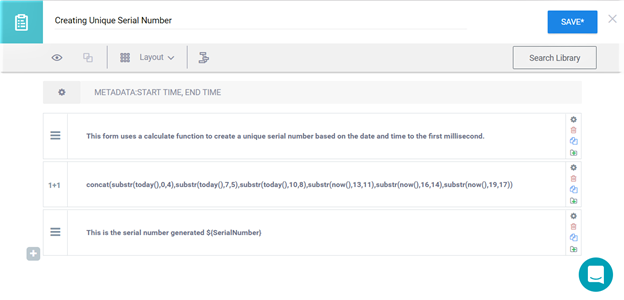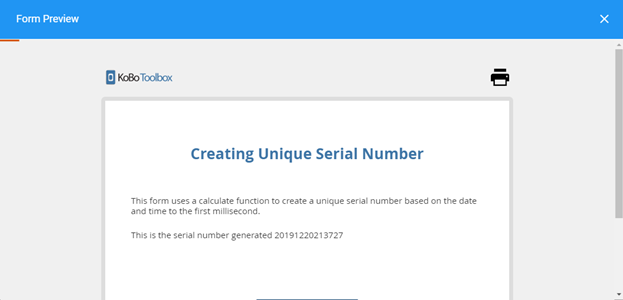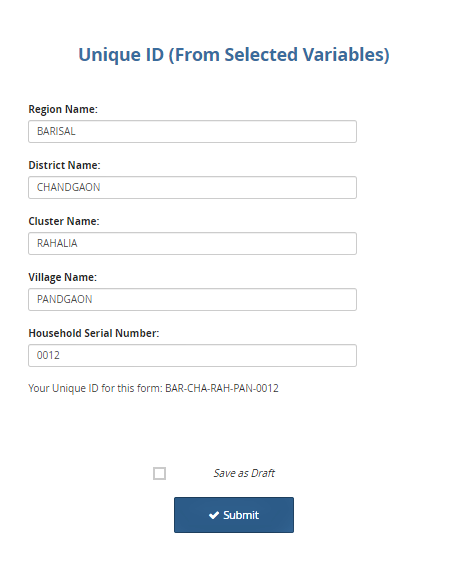# Creating Unique Serial Numbers in Forms¶

Last updated: 11 Jan 2022

There are times you may want to generate a unique serial number for each and every form in a project. This article discusses various workarounds on how to create unique serial numbers using the `calculate` question-type.

## Approach 1: Creating Sequential Unique Serial Numbers Based on Date and Time¶

This method works best with Enketo web forms. It uses a calculation function to create a unique serial number based on the date and time to the first millisecond. Although this method may not meet all your needs, it should give you an illustration of how much you can stretch calculate functions.

Create a `calculate` question type in either the formbuilder or XLSForm and use the formula below.

```concat(substr(today(), 0, 4), substr(today(), 7, 5), substr(today(), 10, 8), substr(now(), 13, 11), substr(now(), 16, 14), substr(now(), 19, 17))
```

The same formula can work as an `integer` question when working in an XLSForm.In the example, when you preview the deployed form in Enketo, you should be able to see the serial number within the note question as shown in the image below:## Approach 2: Creating Unique Serial Numbers from Selected Variables¶

This example shows how to create unique serial numbers from existing, already defined variables in your form by using the `concat()` expression in a `calculate` question type. The example is shown as an XLSForm, but can just as easily be done within the formbuilder.

survey

type

name

label

calculation

text

Q1

Region Name

text

Q2

District Name

text

Q3

Cluster Name

text

Q4

Village Name

text

Q5

Household Serial Number

calculate

Q1_C

substr(\${Q1}, 0, 3)

calculate

Q2_C

substr(\${Q2}, 0, 3)

calculate

Q3_C

substr(\${Q3}, 0, 3)

calculate

Q4_C

substr(\${Q4}, 0, 3)

calculate

ID

concat(\${Q1_C}, ‘-’, \${Q2_C}, ‘-’, \${Q3_C}, ‘-’, \${Q4_C}, ‘-’, \${Q5})

note

note_id

Your Unique ID for this form is: \${ID}

When you preview the example in Enketo web forms, the serial number will be presented within the note question as shown in the image below: